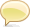# xdmp:set

```xdmp:set(
\$variable as item()*,
\$expr as item()*
) as empty-sequence()```

### Summary

Set the value of a variable to the specified expression. The `xdmp:set` command allows you to introduce changes to the state (side effects) of a query by changing the value of a variable to something other than what it is bound to.

Parameters
\$variable A variable to set.
\$expr A value to set the variable.

### Usage Notes

When a variable is bound to a sequence in a `for` loop, and when that variable is changed by `xdmp:set` in the `return` clause, the change only affects the value for one iteration of the `for` loop at a time; when the next value is sent to the return clause, it is set to the next value in the sequence specified in the `for` clause. The value changes only after the `xdmp:set` call is made.

### Example

```(: set the value of the variable \$x
to 1234 and then print out \$x :)

let \$x := 12
return
(xdmp:set(\$x, 1234), \$x)

=> 1234
```

### Example

```(: set the value of the variable \$x
to 5 and then print out \$x for
each value of \$y :)

for \$x in (1, 2)
for \$y in (\$x, \$x)
return
(\$y, xdmp:set(\$x, 5), \$x)

=> (1, 5, 1, 5, 2, 5, 2, 5)

```

### Example

```(: note the effect on \$z of changing the
value of \$x :)

for \$x in (1, 2)
for \$y in (3,4)
for \$z in (\$x, \$x)
return
(\$z, xdmp:set(\$x, 5))

=> (1, 1, 5, 5, 2, 2, 5, 5)

```

### Example

```(: every time the name of the input node changes,
output the new name  :)

let \$n := ()
for \$i in (<x>1</x>, <x>2</x>, <y>3</y>)
return (
if (name(\$i) eq \$n)
then ()
else (xdmp:set(\$n, name(\$i)), \$n)
, data(\$i)
)

=> (x, 1, 2, y, 3)

```Stack Overflow: Get the most useful answers to questions from the MarkLogic community, or ask your own question.

## CommentsThe commenting feature on this page is enabled by a third party. Comments posted to this page are publicly visible.